# Switching Algebra or Boolean Algebra

Boolean algebra or switching algebra is a system of mathematical logic to perform different mathematical operations in binary system. These are only two elements 1 and 0 by which all the mathematical operations are to be performed. There only three basis binary operations, AND, OR and NOT by which all simple as well as complex binary mathematical operations are to be done. There are many rules in Boolean algebra by which those mathematical operations are done.
In Boolean algebra, the variables are represented by English Capital Letter like A, B, C etc and the value of each variable can be either 1 or 0, nothing else.

Some basic logical Boolean operations-
AND operation,OR operation,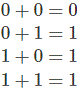Not operation,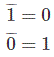Some basic laws for Boolean Algebra,A . 0 = 0 where A can be either 0 or 1.
A . 1 = A where A can be either 0 or 1.
A . A = A where A can be either 0 or 1.
A . Ā = 0 where A can be either 0 or 1.
A + 0 = A where A can be either 0 or 1.
A + 1 = 1 where A can be either 0 or 1.
A + Ā = 1
A + A = A
A + B = B + A where A and B can be either 0 or 1.
A . B = B . A where A and B can be either 0 or 1.
The laws of Boolean algebra are also true for more than two variables like,

### Cumulative Laws for Boolean Algebra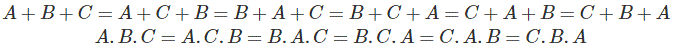### Associative Laws for Boolean Algebra### Distributive Laws for Boolean Algebra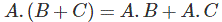### Redundant Literal RuleFrom truth table,

 Inputs Output A B ĀB A + ĀB 0 0 0 0 0 1 1 1 1 0 0 1 1 1 0 1
 Inputs Output A B A+B 0 0 0 0 1 1 1 0 1 1 1 1

From truth table it is proved that,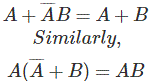### Absorption Laws for Boolean Algebra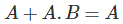Proof from truth table,

 Inputs Output A B AB A+A.B 0 0 0 0 0 1 0 0 1 0 0 1 1 1 1 1

Both A and A+A.B column is same.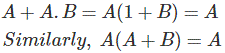Proof from truth table,

 A B A+B A.X(A+B) 0 0 0 0 0 1 1 0 1 0 1 1 1 1 1 1

Both A and A.X or A(A+B) column are same.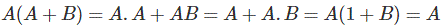De Morgan’s Therem,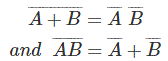Proof from truth table,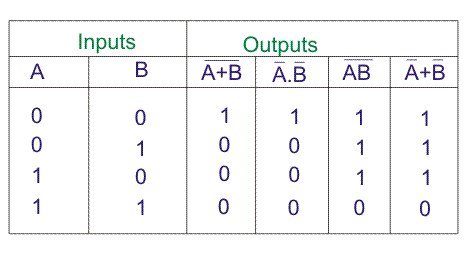## Examples of Boolean Algebra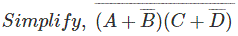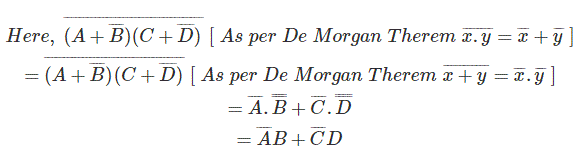These are another method of simplifying complex Boolean expression. In this method we only use three simple steps.

1. Complement entire Boolean expression.
2. Change all ORs to ANDs and all ANDs to ORs.
3. Now, complement each of the variable and get final expression.

By this method,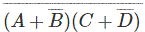will be first complemented, i.e.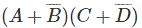.Now, change all (+) to (.) and (.) to (+) i.e.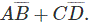Now, complement each of the variable,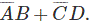This is the final simplified form of Boolean expression,And it is exactly equal to the results which have been come by applying De Morgan Theorem.
Another example,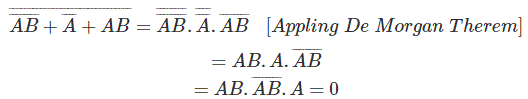By Second Method,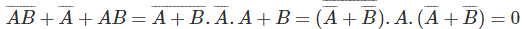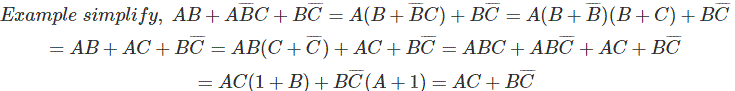Representation of Boolean function in truth table.
Let us consider a Boolean function,Now let us represent the function in truth table.Want To Learn Faster? 🎓
Get electrical articles delivered to your inbox every week.
No credit card required—it’s 100% free.